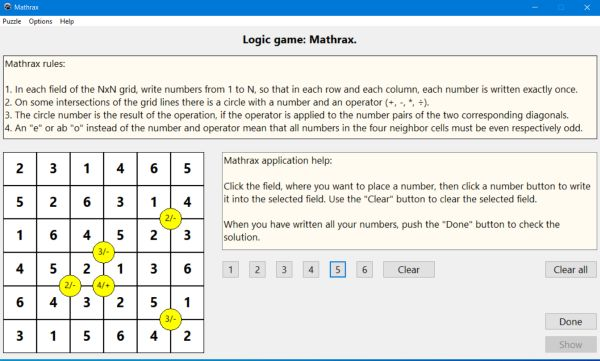# Computing: Free Pascal Programming

## Logic number puzzle: Mathrax.

Description: Mathrax is a puzzle game with numbers to be placed onto a grid. The rules of the game are as follows:
1. In each field of the NxN grid, write numbers from 1 to N, so that in each row and each column, each number is written exactly once.
2. On some intersections of the grid lines there is a circle with a number and an operator (+, -, *, ÷).
3. The circle number is the result of the operation, if the operator is applied to the number pairs of the two corresponding diagonals.
4. An "e" or ab "o" instead of the number and operator mean that all numbers in the four neighbor cells must be even respectively odd.
The game is a typical logic game: In order to find the correct solution of the puzzle, you have to explore the numbers already written, and by logical thinking (concluding from what you know, that a given field must be a given number, or on the contrary, can't be a given number), determine, which fields have to contain which numbers. The Mathrax application comes with 3 different grid sizes and two different difficulty levels.
The game is played with the mouse: Click the field, where you want to write a number, then click one of the number buttons to write the corresponding number or Clear to remove the number from the actually selected field.

Free Pascal features: Creating objects during run-time. Changing the color of shapes and the caption and of static-texts during run-time. Using buttons to insert characters into a given control's caption. Two-dimensional arrays, arrays of records (classic Pascal).

Screenshot: